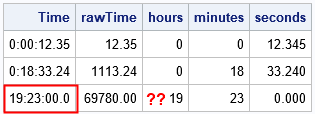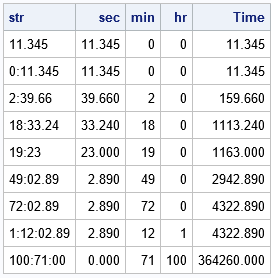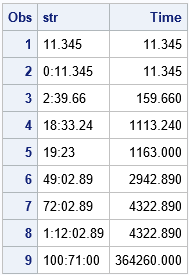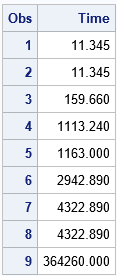An informat helps you read data into a SAS data set. SAS supports more than 100 informats. The most common informats are related to dates and times and make it easy to read an input string such as 28JAN2001 and convert it to a SAS date such as 15003. Yet no matter how many built-in informats SAS provides, programmers will inevitably encounter data that are difficult to read. That is why PROC FORMAT in SAS enables you to create a custom informat. A custom informat enables you to process input strings in whatever way is most convenient for your application. This article shows how to create a custom informat in SAS in three easy steps:

1. Write DATA step code that parses an input string and converts it into a form that is more convenient to work with.
2. Use PROC FCMP to define a function that implements the same string-to-value conversion. Often you can cut and paste from your DATA step program into PROC FCMP.
3. Use the INVALUE statement in PROC FORMAT to define a custom informat.

Technically, you can skip Step 1, but I find it much easier to develop and debug a program in the DATA step because I can use the PUT statement or PROC PRINT to look at intermediate results.

### A motivating example: Convert durations into seconds

While writing a recent article about how to visualize race times, I researched ways to read times into SAS. For time-of-day data, you can use the TIMEw. or ANYDTTME. informats to read a string in the form HH:MM:SS and convert it to a SAS time value. For example, the time "1:23:45" (AM) is converted to the number of seconds since midnight, which is 5025.

Race times are slightly different because they represents durations of time. Short races such as a 5K are typically recorded in minutes, and sprints are recorded in seconds. Both times contain fractions of seconds, so the data is typically represented as SS.fff or MM:SS.ff. You can use the HHMMSS. informat to read these data and store them as seconds. The following DATA step reads a sprint time (12.345 s) and a 5K time (18:33.24) into a SAS time variable. You can use the HOUR, MINUTE, and SECOND functions to make sure the data are read correctly:

```/* You can use the HHMMSS informat to read durations into SAS */ data RaceTimes; input Time HHMMSS.; /* read duration = length of time */ format Time TIME10.3; /* there is no HHMMSS. format, so use TIME. format */ rawTime = Time; hours = hour(Time); /* check the results by print hour, min, and sec */ minutes = minute(Time); seconds = second(Time); datalines; 12.345 18:33.24 19:23 ;   proc print noobs; run;```The only problem with the HHMMSS. informat is that it reads the string 19:23 as "19 hours, 23 minutes," whereas I prefer "19 minutes, 23 seconds." (It's an ambiguous case. For clarity, the string could be entered as 0:19:23 or 19.23.0.) If a SAS informat does not interpret strings the way you prefer, you can write your own SAS informat. The main challenge is writing DATA step code that parses strings such as "12.345" and "18:33.24" and computes the number of seconds that each string represents. The next section presents a DATA step program that converts these strings to seconds. You can use the program to define a new informat that parses race-time data.

### Step 1: Write a DATA step that converts a string into a number

This article is about how to create an informat, not about how to parse strings in SAS, so I'll let the comments in the program speak for themselves. Basically, the following program reads from the right side of a string until it encounters a colon (or the beginning of the string). It extracts the string following the colon and converts it to a number, which is the number of seconds. It repeats the process to find the number of minutes (if any) and the number of hours (if any). It then converts the sum of the hours, minutes, and seconds to seconds and stores it in a variable named Time.

```/* Parse strings. Obtain time, in seconds, for durations written in the following forms: SS.fff MM:SS.fff HHH:MM:SS.fff */ data RaceTimes(drop= i j z); length str \$13; /* HHH.MM.SS.fff */ input str; /* read raw string */ s=0; m=0; h=0; /* read seconds :SS.fff */ i = find(str, ':', -length(str)+1); /* find colon from right */ z = substr(str, i+1); /* extract seconds */ s = input(z, best6.); /* convert to number */ /* read minutes :MM: */ if i > 0 then do; j = find(str, ':', -(i-1)); /* find colon from right */ z = substr(str, j+1, i-j-1); /* extract minutes */ m = input(z, best6.); /* convert to number */ /* read hours HHH:, if any */ if j > 0 then do; z = substr(str, 1, j-1); /* extract hours */ h = input(z, best6.); /* convert to number */ end; end; Time = 3600*h + 60*m + s; /* convert to seconds */ datalines; 11.345 0:11.345 2:39.66 18:33.24 19:23 49:02.89 72:02.89 1:12:02.89 100:71:00 ;   proc print data=RaceTimes noobs; format time 10.3; run;```The DATA step parses each string and converts it to a time, in seconds. The output shows the results. This table is the same as you would get by using the HHMMSS. informat to read the data, except that the string 19:23 is now interpreted as "19 minutes, 23 seconds."

Notice that you can see the intermediate expressions such as s, m, and h. This is useful when you are trying to debug the program.

### Step 2: Use PROC FCMP to define a function

You can use PROC FCMP to define a custom function in Base SAS. This example defines a function named Duration, which takes a string as an input argument. Copy and paste the DATA step code and return the Time variable. You can use the OPTIONS CMPLIB= system option to tell SAS where to find the Duration function. You can then call the new function from the DATA step, as follows:

```proc fcmp outlib=work.functions.MathFuncs; function Duration(str \$); s=0; m=0; h=0; /* read seconds :SS.fff */ i = find(str, ':', -length(str)+1); /* find colon from right */ z = substr(str, i+1); /* extract seconds */ s = input(z, best6.); /* convert to number */ /* read minutes :MM: */ if i > 0 then do; j = find(str, ':', -(i-1)); /* find colon from right */ z = substr(str, j+1, i-j-1); /* extract minutes */ m = input(z, best6.); /* convert to number */ /* read hours, if any */ if j > 0 then do; z = substr(str, 1, j-1); /* extract hours */ h = input(z, best6.); /* convert to number */ end; end; Time = 3600*h + 60*m + s; /* convert to seconds */ return( Time ); endsub; quit;   options cmplib=work.functions; /* where to find Duration() function */ data RaceTimes2; length str \$13; input str; /* call Duration function to convert string to a time value */ Time = Duration(str); datalines; 11.345 0:11.345 2:39.66 18:33.24 19:23 49:02.89 72:02.89 1:12:02.89 100:71:00 ;```### Step 3: Use PROC FORMAT to define a custom informat

I have written several articles about how to use PROC FORMAT to create your own custom formats in SAS. The process of creating an informat is similar, but because parsing strings is complicated it is often best to write the code into an FCMP function and then define the informat in terms of the function. This is done in the following call to PROC FORMAT, which uses the INVALUE statement to define an informat named DURATION.:

```/* define the DURATION. informat */ proc format; invalue duration (default=13) other=[Duration()]; run;   /* use new DURATION informat to read duration times into SAS */ data RaceTimes3; input Time : DURATION.; datalines; 11.345 0:11.345 2:39.66 18:33.24 19:23 49:02.89 72:02.89 1:12:02.89 100:71:00 ;   proc print data=RaceTimes3; format Time 10.3; run;```Success! The program uses the DURATION. informat to read strings of the form "SS.fff", "MM:SS.fff", and "HHH:MM:SS.fff". It converts the strings to seconds. You can use the statement FORMAT Time TIME10.3 in PROC PRINT if you want to format the seconds as hours, minutes, and seconds.

In summary, you can use the INVALUE statement in PROC FORMAT to create a custom informat in SAS. An informat enables you to read a string or pattern and convert it to a value that is easier to work with. Although SAS supports the HHMMSS. informat for reading strings that represent durations of time, I chose to create my own informat that converts race times to seconds. You can use PROC FCMP to define a function that parses a string and returns a numerical value. You can then use PROC FORMAT to define the informat, which calls the FCMP function.

The post Create a custom informat in SAS appeared first on The DO Loop.